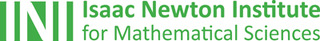## The Isaac Newton Institute: Creating eureka momentsOne of the most exciting places in the mathematical world is the Isaac Newton Institute for Mathematical Sciences (INI), an international research centre and our neighbour here on the University of Cambridge's maths campus.
The INI attracts leading mathematical scientists from all over the world, and is open to all. We are proud to be collaborating with the INI to bring the cutting edge mathematics that is being done there to the general public. The following content is part of this collaboration.

Maths in a Minute: Turbulence and the Reynolds number

How does a smooth flow suddenly become turbulent? The Reynolds number helps understand the transition.

In this year's advent calendar we celebrate our collaboration with the Isaac Newton Institute for Mathematical Sciences.

Maths in a Minute: Fluid dynamics and the Euler equations

How does water, or indeed any fluid, move? The Euler equations let us look beneath the surface and mark the beginning of modern fluid dynamics.

Maths in a minute: Asymptotic expansions

Approximating a function with a divergent series.

The power of small things: Resurgent asymptotics

If you ignore exponentially small terms they can come back to bite you.

How a question about rainbows led to an entire field of mathematics.

The unity of mathematics: a meeting in honour of Sir Michael Atiyah

Mathematicians from across maths and physics and around the world gathered to celebrate Sir Michael Atiyah.

Black Heroes of Mathematics 2021

This inspiring conference featured speakers from Africa, the US, the Caribbean and the UK. Here are some highlights.

Keeping people safe at large events

How can sporting events, concerts, or festivals be kept safe in the face of rising COVID-19 infections?

Maths in a Minute: Category theory

A quick introduction to an abstract concept that's useful in anything from genetics to cosmology.

• Want facts and want them fast? Our Maths in a minute series explores key mathematical concepts in just a few words.